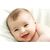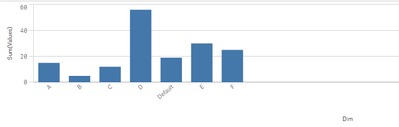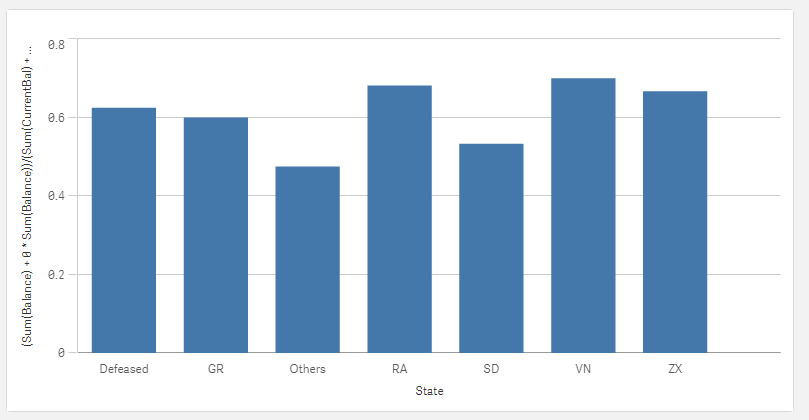# New to Qlik Sense

If you’re new to Qlik Sense, start with this Discussion Board and get up-to-speed quickly.

Announcements
Read about the latest Qlik Community enhancements on the Community News blog!
cancel
Showing results for
Did you mean:Creator II

## Bar Chart --> Top 5 and default dimension value in QlikSense

Hi All, @sunny_talwar

Can anyone help me out with this scenario.

I have a Bar Chart and have to limit the values.1. I have to show top 5 --> sum(values) among A,B,C,D,E,F exclude 'Default'

2. I have to show sum(values) for 'Default' value always in Bar Chart.

3. Others --> This includes the values which are apart from Top 5 and default.

Thanks & Regards,

Abhijith

Labels (1)
• ### Qlik Sense

1 Solution

Accepted SolutionsMVP

try blow

``````=If(aggr(Rank( (sum({<State-={'Defeased'} >} Balance)/sum({<State-={'Defeased'} >}CurrentBal))),State)<=5 ,
sum(Balance)/sum(CurrentBal),
if( State='Defeased',
rangemin((sum(Balance)/sum(CurrentBal)),-1),
rangemin((sum(Balance)/sum(CurrentBal)),0)))``````

35 RepliesMVP

May be try this as your calculated dimension

``````Aggr(
If(Rank(Sum({<Dim -= {'Default'}>}values)) < 6 or Dim = 'Default', Dim, 'Other')
, Dim)``````

``Sum({<Dim += {'Default'}>} values)``Creator II
Author

Thanks for sharing the logic.

Now i am finding with the sorting. I need to sort with 3 different conditions.

1. Top 5 in desc order.

2. then Others.

3. last value always it should 'default'MVP

Are you able to share a sample where we can see this? Or you can play around with an expression will give the max value to what you want to see as the first and lowest to default.Creator II
Author

So, this the dimension expression:

This is my Dimension.

=if(State='Defeased',State,
If(Aggr(Rank( (sum({<State-={'Defeased'} >} [Balance])/sum({<State-={'Defeased'} >}[Balance (\$)))),State)<=5, State,'Others'))

This is the expression.

Sum(Balance)/sum(Current Bal)

I need to sort on 3 conditions:

1: Top 5 State on desc order.

2: show Other

3: DefeasedMVP

Can you share a sample app or some sample data where we can test this out?Creator II
Author

I have to show the  top 5 states, apart from defeased.

Thanks,MVP

Is this what you want?Dimension

``````=Aggr(
If(State = 'Defeased', State,
If(Rank((Sum({<State-={'Defeased'}>} Balance) + 0 * Sum({<State-={'Defeased'}>} Balance))/(Sum({<State-={'Defeased'}>} CurrentBal) + 0 * Sum({1<State-={'Defeased'}>} CurrentBal))) <= 5, State, 'Others'))
, State)``````

Expression

``(Sum(Balance) + 0 * Sum(Balance))/(Sum(CurrentBal) + 0 * Sum({1} CurrentBal))``MVP

see the attachedCreator II
Author

Thanks for sharing the solution. The output should be sorted for Top 5 states first, then others, last should be defeased.

Thanks for sharing the solution. I have a doubt. Cold you please tell me , why the last condition with -100 and -50 are used for sorting.

=If(aggr(Rank( (sum({<State-={'Defeased'} >} Balance)/sum({<State-={'Defeased'} >}CurrentBal))),State)<=5 ,
sum(Balance)/sum(CurrentBal),
if( State='Defeased',
(sum(Balance)/sum(CurrentBal))-100,
(sum(Balance)/sum(CurrentBal))-50))

Thanks,Tags
Community Browser